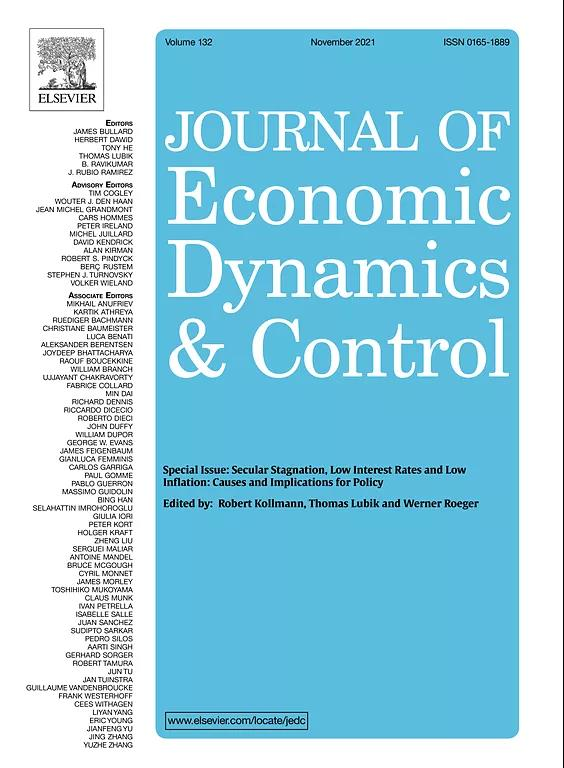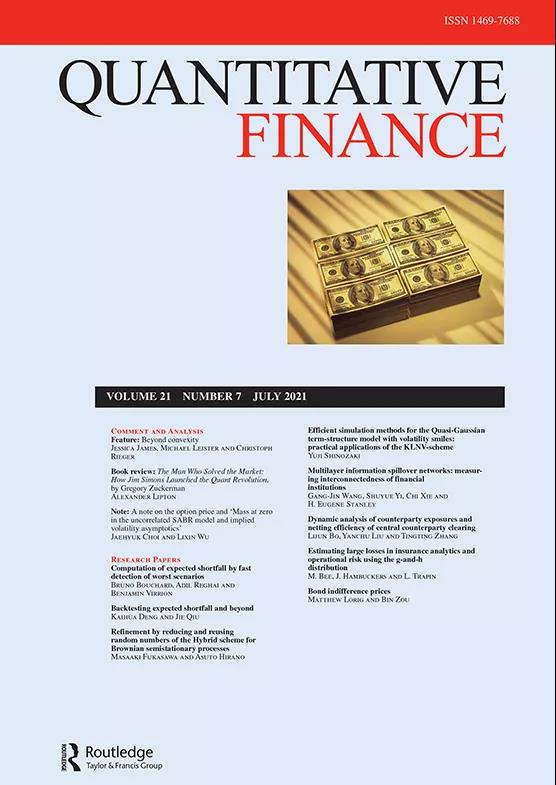Professor Choi's research: Recent advances on the stochastic-alpha-beta-rho (SABR) model
The Black-Scholes (BS) model has been the standard option pricing model in the past few decades. But the model fails to explain non-constant implied volatilities across strike prices, a phenomenon called volatility smile, which has been clearly observed in the options market since the Black Monday stock market crash in 1987. Numerous attempts have been made to replace or improve the BS framework, which has been a significant part of mathematical finance research.

One of the successful approaches to accurately model the volatility smile is to make volatility stochastic rather than constant. Among several stochastic volatility (SV) models, the stochastic-alpha-beta-rho (SABR) model proposed by a renowned quantitative analyst, Patrick Hagan, has been widely used in the financial industry. The SABR model is intuitive and parsimonious. It also provides a flexible choice of leverage effect, the correlation between the volatility and spot price. Due to complexity, the exact option price, similar to the BS formula, is not available for the SABR model. Hagan instead provided an approximation formula for the equivalent BS volatility so that traders could quickly convert to the option price. However, the approximation formula should be used with caution because the error often grows to a non-negligible size, breaking the no-arbitrage condition. As such, There have been many attempts to find a more accurate yet efficient method to improve Hagan's approximation.

To this end,Prof. Choi and his coauthor, Prof. Wu from HKUST, recently published two papers: "The equivalent constant-elasticity-of-variance (CEV) volatility of the stochastic-alpha-beta-rho (SABR) model" in the Journal of Economic Dynamics and Control and "A note on the option price and Mass at zero in the uncorrelated SABR model and implied volatility asymptotics" in Quantitative Finance.Both papers focus on using the constant-elasticity-of-variance (CEV) model, which is the fixed volatility limit of the SABR model. In the first paper, the authors derived the equivalent CEV volatility of the SABR model instead of the equivalent BS volatility. This new approach reduces error effectively as a control variate method because of the close connection between CEV and SABR models. Moreover, mapping to the CEV volatility indirectly imposes absorbing boundary conditions at zero strikes and thus provides small-time asymptotics for mass at zero, consistent with the literature.In the second paper, the authors approximate the SABR model as a finite mixture of the CEV models with different spot and volatility values, using the idea borrowed from the method of Gulisashvili, Horvath, and Jacquier (2018). Therefore, the SABR option price is quickly obtained as the weighted sum of the CEV option prices. Although the approach is limited to the special uncorrelated case, the new finite-mixture option-pricing method is accurate and arbitrage-free across all strike prices. Numerical results demonstrate that the two new methods are more accurate with less or no arbitrage, showing a good promise to replace Hagan's formula in the finance industry.LATEST NEWS